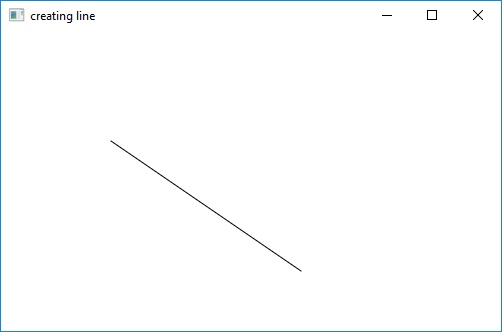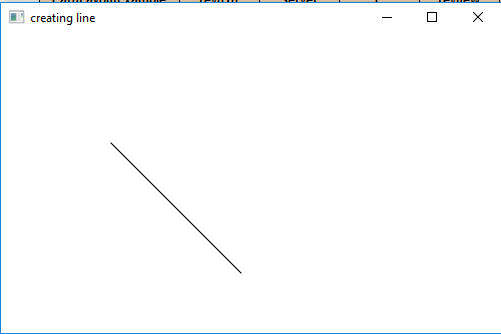Related Articles
JavaFX | Line with examples
• Last Updated : 06 Aug, 2018

Line is a part of JavaFX. The Line class represents a line in a 2D space.

Constructor for the class:

1. Line(): Creates a new instance for line
2. Line(double startX, double startY, double endX, double endY): Creates a new Line with specified staring and ending point

Commonly Used Methods:

Method Explanation
getEndX() returns the x coordinate for the end point
getEndY() returns the y coordinate for the end point
getStartX() returns the x coordinate for the start point
getStartY() returns the y coordinate for the start point
setEndX(double value) sets the x coordinate for the end point
setEndY(double value) sets the y coordinate for the end point
setStartX(double value) sets the x coordinate for the start point
setStartY(double value) sets the y coordinate for the start point

Below programs illustrate the Line class:

1. Java program to create a line with starting and ending coordinates passed as arguments: This program creates a Line indicated by the name line( start point and the end point is passed as arguments). The Line will be created inside a scene, which in turn will be hosted inside a stage. The function setTitle() is used to provide title to the stage. Then a Group is created, and the line is attached. The group is attached to the scene. Finally, the show() method is called to display the final results.

 `// Java program to create a line with starting ` `// and ending coordinates passed as arguments ` `import` `javafx.application.Application; ` `import` `javafx.scene.Scene; ` `import` `javafx.scene.shape.DrawMode; ` `import` `javafx.scene.layout.*; ` `import` `javafx.event.ActionEvent; ` `import` `javafx.scene.shape.Line; ` `import` `javafx.scene.control.*; ` `import` `javafx.stage.Stage; ` `import` `javafx.scene.Group; ` ` `  `public` `class` `line_0 ``extends` `Application { ` ` `  `    ``// launch the application ` `    ``public` `void` `start(Stage stage) ` `    ``{ ` `         `  `        ``// set title for the stage ` `        ``stage.setTitle(``"creating line"``); ` ` `  `        ``// create a line ` `        ``Line line = ``new` `Line(``10``.0f, ``10``.0f, ``200``.0f, ``140``.0f); ` ` `  `        ``// create a Group ` `        ``Group group = ``new` `Group(line); ` ` `  `        ``// translate the line to a position ` `        ``line.setTranslateX(``100``); ` `        ``line.setTranslateY(``100``); ` ` `  `        ``// create a scene ` `        ``Scene scene = ``new` `Scene(group, ``500``, ``300``); ` ` `  `        ``// set the scene ` `        ``stage.setScene(scene); ` ` `  `        ``stage.show(); ` `    ``} ` ` `  `    ``// Main Method ` `    ``public` `static` `void` `main(String args[]) ` `    ``{ ` `        ``// launch the application ` `        ``launch(args); ` `    ``} ` `} `

Output:2. Java program to create a line with starting and ending coordinates set using function setStartX(), setStartY()setEndX(), setEndY() function: This program creates a Line indicated by the name line(start point and end point is set using setEndX(), setEndY(), setStartX(), setStartY() function). The Line will be created inside a scene, which in turn will be hosted inside a stage. The function setTitle() is used to provide title to the stage. Then a Group is created, and the line is attached.The group is attached to the scene. Finally, the show() method is called to display the final results.

 `// Java program to create a line with starting  ` `// and ending coordinates set using function  ` `// setStartX(), setStartY() setEndX(), ` `// setEndY() function ` `import` `javafx.application.Application; ` `import` `javafx.scene.Scene; ` `import` `javafx.scene.shape.DrawMode; ` `import` `javafx.scene.layout.*; ` `import` `javafx.event.ActionEvent; ` `import` `javafx.scene.shape.Line; ` `import` `javafx.scene.control.*; ` `import` `javafx.stage.Stage; ` `import` `javafx.scene.Group; ` ` `  `public` `class` `line_1 ``extends` `Application { ` ` `  `    ``// launch the application ` `    ``public` `void` `start(Stage stage) ` `    ``{ ` `        ``// set title for the stage ` `        ``stage.setTitle(``"creating line"``); ` ` `  `        ``// create a line ` `        ``Line line = ``new` `Line(); ` ` `  `        ``// set staring position ` `        ``line.setStartX(``10``.0f); ` `        ``line.setStartY(``10``.0f); ` ` `  `        ``// set ending position ` `        ``line.setEndX(``140``.0f); ` `        ``line.setEndY(``140``.0f); ` ` `  `        ``// create a Group ` `        ``Group group = ``new` `Group(line); ` ` `  `        ``// translate the line to a position ` `        ``line.setTranslateX(``100``); ` `        ``line.setTranslateY(``100``); ` ` `  `        ``// create a scene ` `        ``Scene scene = ``new` `Scene(group, ``500``, ``300``); ` ` `  `        ``// set the scene ` `        ``stage.setScene(scene); ` ` `  `        ``stage.show(); ` `    ``} ` ` `  `    ``// Main Method ` `    ``public` `static` `void` `main(String args[]) ` `    ``{ ` `         `  `        ``// launch the application ` `        ``launch(args); ` `    ``} ` `} `

Output:Note: The above programs might not run in an online IDE. Please use an offline compiler.

My Personal Notes arrow_drop_up
Recommended Articles
Page :